Question 20

Evaluate: Definite Integration -1 → 1  ∫ (x + |x| + 1) / (x + 2|x| + 1)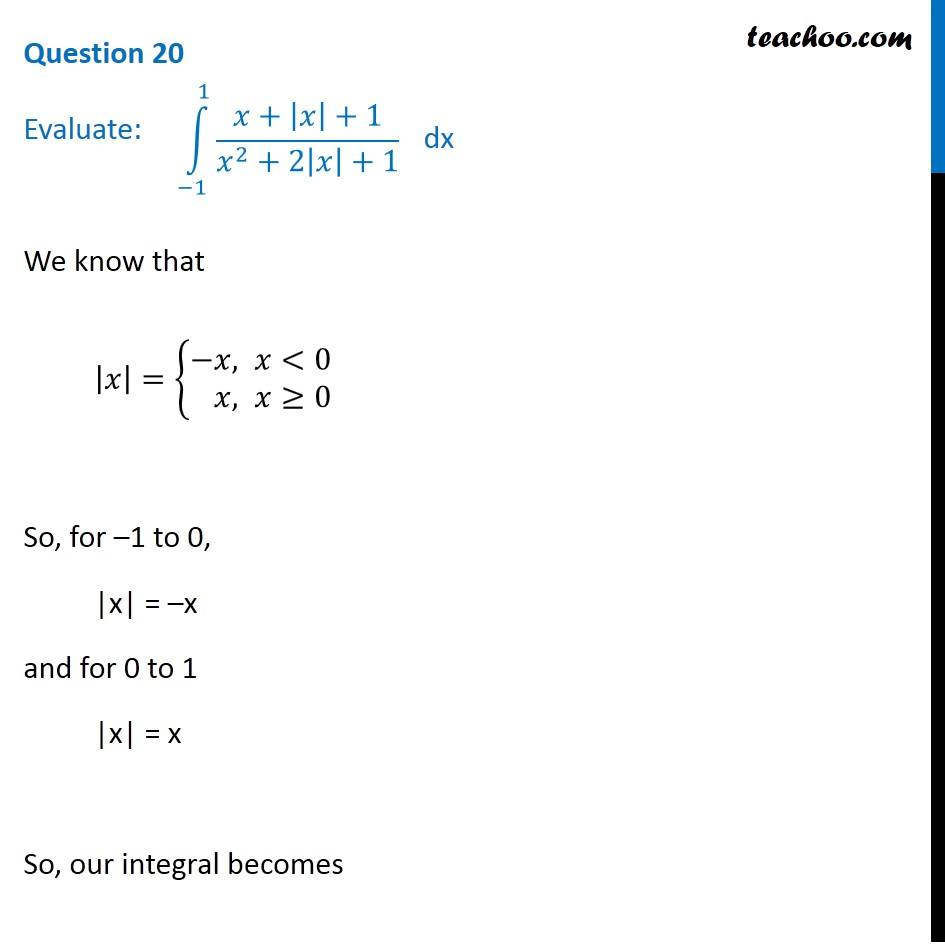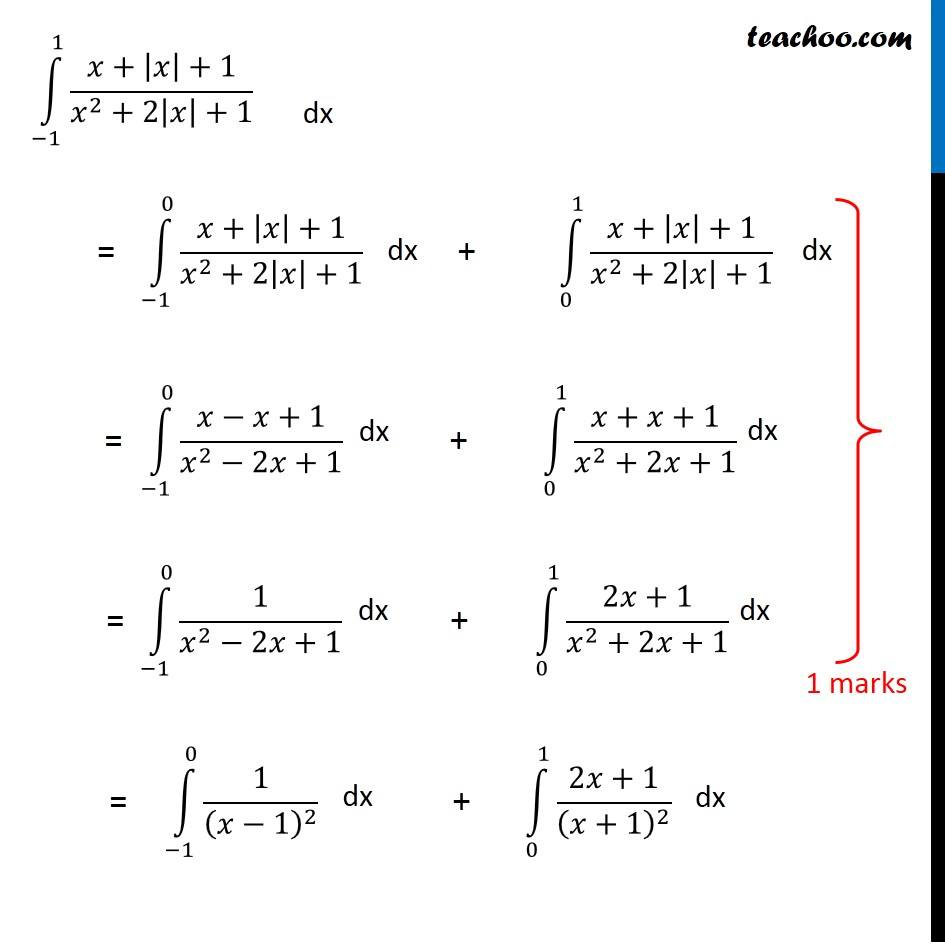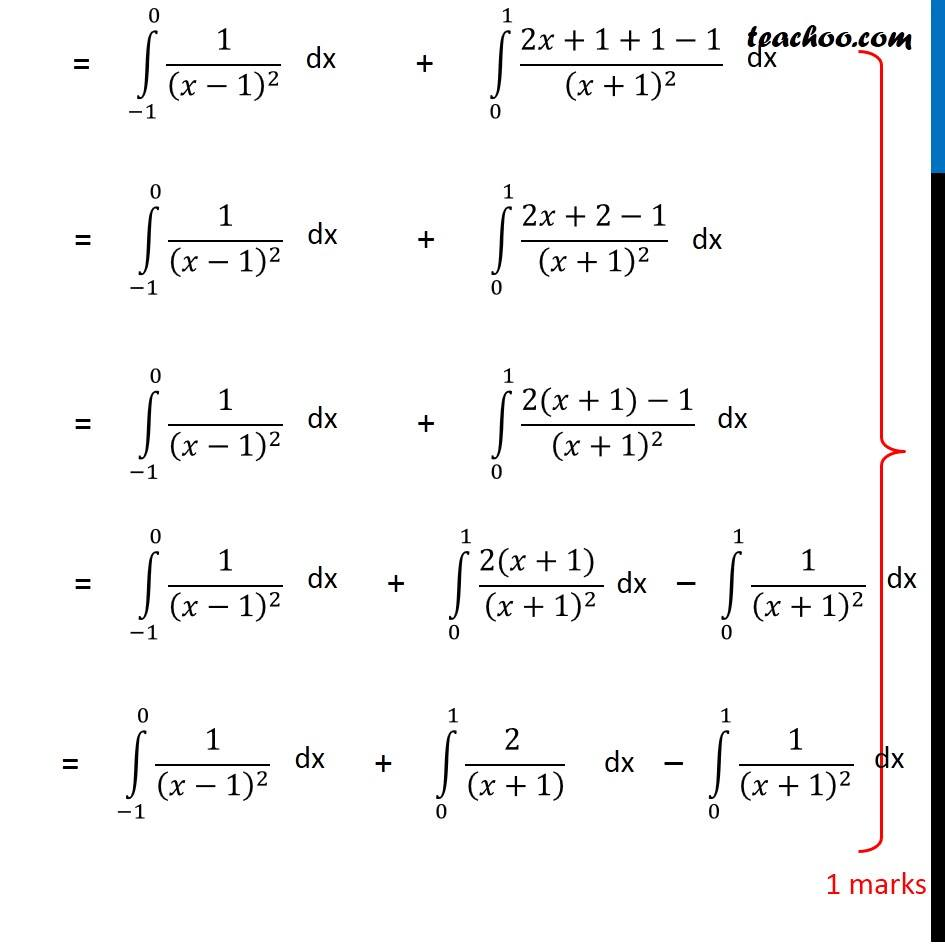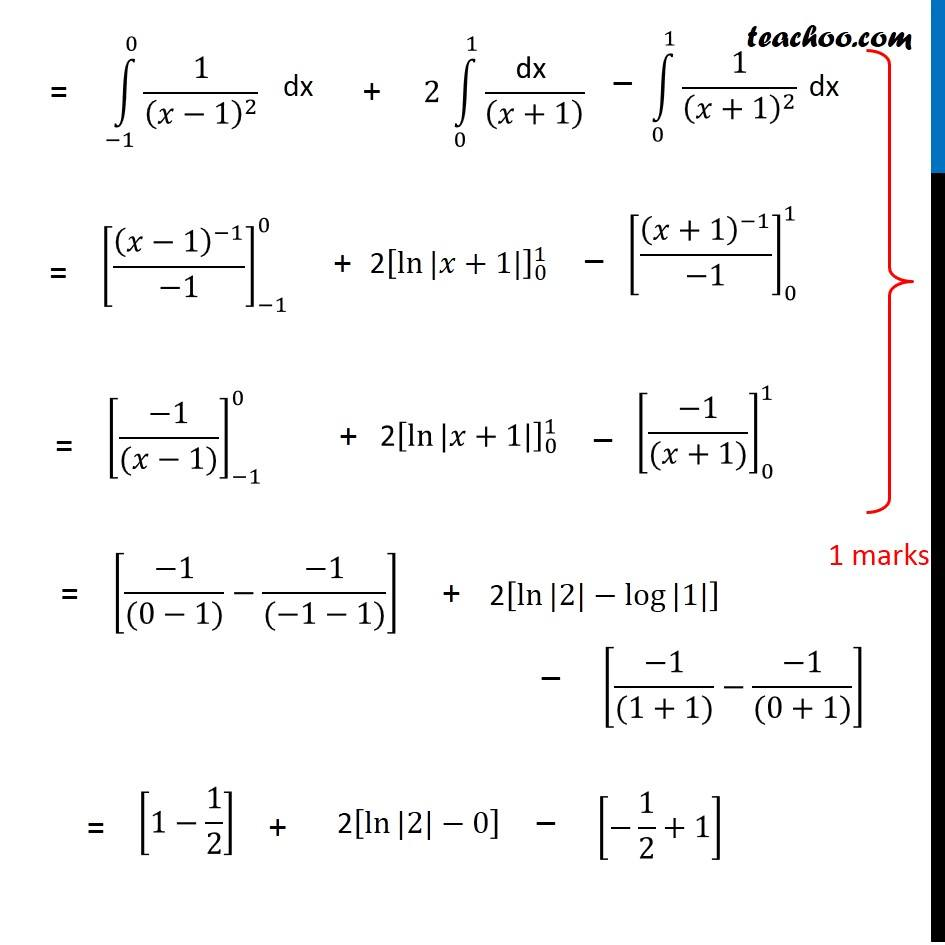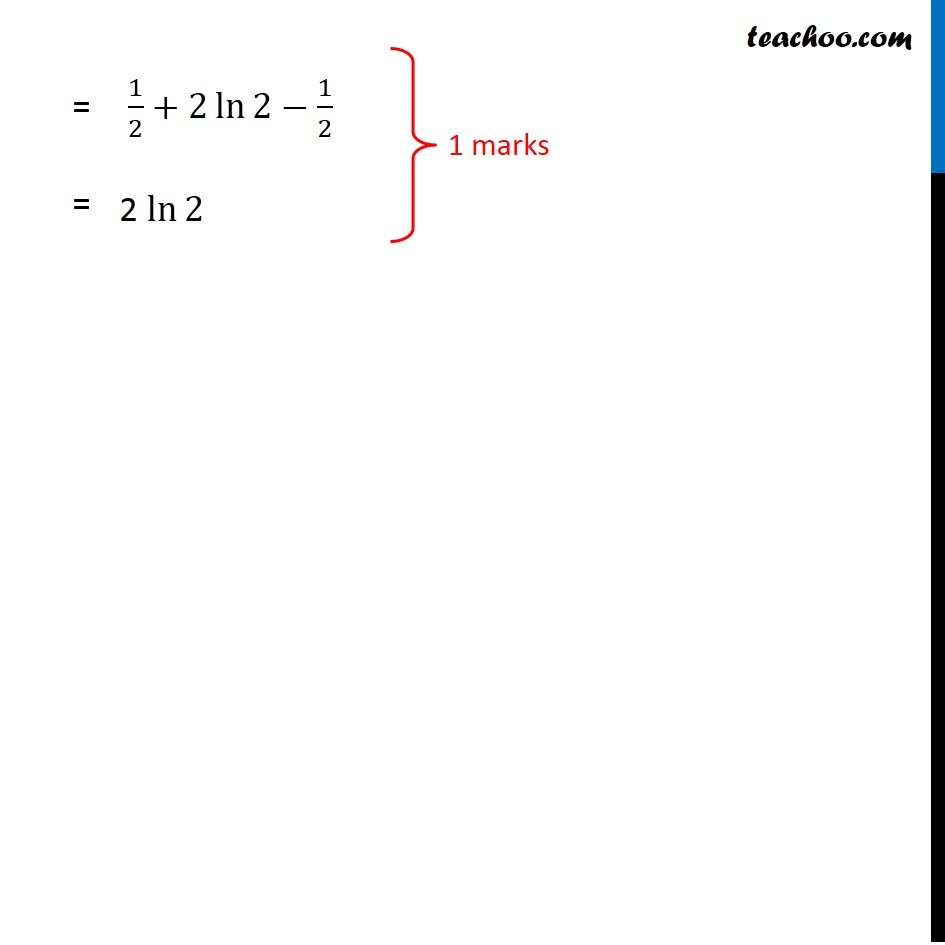1. Class 12
2. Solutions of Sample Papers and Past Year Papers - for Class 12 Boards
3. CBSE Class 12 Sample Paper for 2019 Boards

Transcript

Question 20 Evaluate: ∫1_(−1)^1▒(𝑥+|𝑥|+1)/(𝑥^2+2|𝑥|+1) We know that |𝑥|={█(−&𝑥, 𝑥<0@&𝑥, 𝑥≥0)┤ So, for –1 to 0, |x| = –x and for 0 to 1 |x| = x So, our integral becomes ∫1_(−1)^1▒(𝑥+|𝑥|+1)/(𝑥^2+2|𝑥|+1) ∫1_(−1)^0▒(𝑥+|𝑥|+1)/(𝑥^2+2|𝑥|+1) ∫1_0^1▒(𝑥+|𝑥|+1)/(𝑥^2+2|𝑥|+1) ∫1_(−1)^0▒(𝑥−𝑥+1)/(𝑥^2−2𝑥+1) ∫1_0^1▒(𝑥+𝑥+1)/(𝑥^2+2𝑥+1) ∫1_(−1)^0▒1/(𝑥^2−2𝑥+1) ∫1_0^1▒(2𝑥+1)/(𝑥^2+2𝑥+1) ∫1_(−1)^0▒1/(𝑥−1)^2 ∫1_0^1▒(2𝑥+1)/(𝑥+1)^2 ∫1_(−1)^0▒1/(𝑥−1)^2 ∫1_0^1▒(2𝑥+1+1−1)/(𝑥+1)^2 ∫1_(−1)^0▒1/(𝑥−1)^2 ∫1_0^1▒(2𝑥+2−1)/(𝑥+1)^2 ∫1_(−1)^0▒1/(𝑥−1)^2 ∫1_0^1▒(2(𝑥+1)−1)/(𝑥+1)^2 ∫1_(−1)^0▒1/(𝑥−1)^2 ∫1_0^1▒(2(𝑥+1) )/(𝑥+1)^2 ∫1_0^1▒1/(𝑥+1)^2 ∫1_(−1)^0▒1/(𝑥−1)^2 ∫1_0^1▒(2 )/((𝑥+1) ) ∫1_0^1▒1/(𝑥+1)^2 ∫1_(−1)^0▒1/(𝑥−1)^2 2 ∫1_0^1▒"dx " /((𝑥+1) ) ∫1_0^1▒1/(𝑥+1)^2 [(𝑥−1)^(−1)/(−1)]_(−1)^0 2[ln⁡〖|𝑥+1|〗 ]_0^1 [(𝑥+1)^(−1)/(−1)]_0^1 [(−1)/((𝑥−1))]_(−1)^0 "2" [ln⁡〖|𝑥+1|〗 ]_0^1 [(−1)/((𝑥+1))]_0^1 [(−1)/((0−1))−(−1)/((−1−1))] 2[ln⁡〖|2|〗−log⁡〖|1|〗 ] [(−1)/((1+1))−(−1)/((0+1))] [1−1/2] 2[ln⁡〖|2|〗−0] [−1/2+1] 1/2+2 ln⁡2−1/2 2 ln⁡2

CBSE Class 12 Sample Paper for 2019 Boards

Class 12
Solutions of Sample Papers and Past Year Papers - for Class 12 Boards